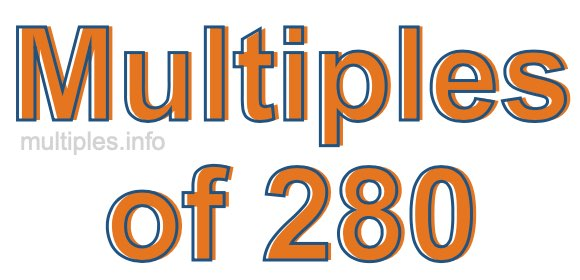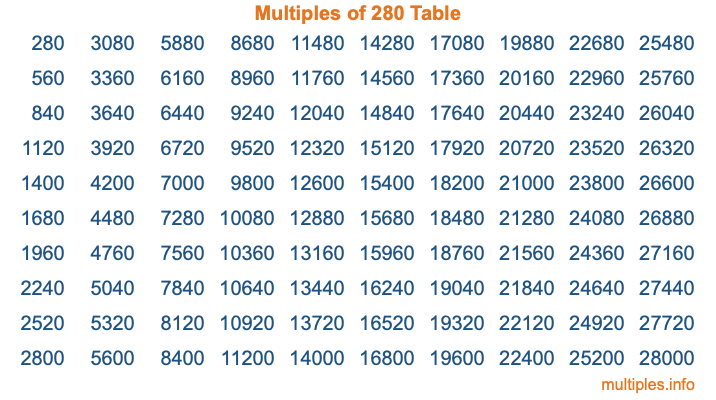Multiples of 280Welcome to the Multiples of 280 page. Here we will first teach you everything you will ever need to know about the multiples of 280, and then give you a study guide summary of everything we taught you to make sure you remember it all. Use this page to look up facts and learn information about the multiples of 280. This page will make you a multiples of two hundred eighty expert!

Definition of Multiples of 280
Multiples of 280 are all the numbers that when divided by 280 equal an integer. Each of the multiples of 280 are called a multiple. A multiple of 280 is created by multiplying 280 by an integer.

Therefore, to create a list of multiples of 280, you start with 1 multiplied by 280, then 2 multiplied by 280, then 3 multiplied by 280, and so on for as long as you want. Thus, the list of the first five multiples of 280 is 280, 560, 840, 1120, and 1400. To see a larger list of multiples of 280, see the printable image of Multiples of 280 further down on this page. We also have a category where you can choose any nth multiple of 280.

Multiples of 280 Checker
The Multiples of 280 Checker below checks to see if any number of your choice is a multiple of 280. In other words, it checks to see if there is any number (integer) that when multiplied by 280 will equal your number. To do that, we divide your number by 280. If the the quotient is an integer, then your number is a multiple of 280.

Is  a multiple of 280?

Least Common Multiple of 280 and ...
A Least Common Multiple (LCM) is the lowest multiple that two or more numbers have in common. This is also called the smallest common multiple or lowest common multiple and is useful to know when you are adding our subtracting fractions. Enter one or more numbers below (280 is already entered) to find the LCM.

Check out our LCM Calculator if you need more details about the Least Common Multiple or if you need the LCM for different numbers for adding and subtraction fractions.

nth Multiple of 280
As we stated above, 280 is the first multiple of 280, 560 is the second multiple of 280, 840 is the third multiple of 280, and so on. Enter a number below to find the nth multiple of 280.

th multiple of 280

Multiples of 280 vs Factors of 280
280 is a multiple of 280 and a factor of 280, but that is where the similarities end. All postive multiples of 280 are 280 or greater than 280. All positive factors of 280 are 280 or less than 280.

Below is the beginning list of multiples of 280 and the factors of 280 so you can compare:

Multiples of 280: 280, 560, 840, 1120, 1400, etc.

Factors of 280: 1, 2, 4, 5, 7, 8, 10, 14, 20, 28, 35, 40, 56, 70, 140, 280

As you can see, the multiples of 280 are all the numbers that you can divide by 280 to get a whole number. The factors of 280, on the other hand, are all the whole numbers that you can multiply by another whole number to get 280.

It's also interesting to note that if a number (x) is a factor of 280, then 280 will also be a multiple of that number (x).

Multiples of 280 vs Divisors of 280
The divisors of 280 are all the integers that 280 can be divided by evenly. Below is a list of the divisors of 280.

Divisors of 280: 1, 2, 4, 5, 7, 8, 10, 14, 20, 28, 35, 40, 56, 70, 140, 280

The interesting thing to note here is that if you take any multiple of 280 and divide it by a divisor of 280, you will see that the quotient is an integer.

Multiples of 280 Table
Below is an image of the first 100 multiples of 280 in a table. The table is in chronological order, column by column. The first column has the first ten multiples of 280, the second column has the next ten multiples of 280, and so on.The Multiples of 280 Table is also referred to as the 280 Times Table or Times Table of 280. You are welcome to print out our table for your studies.

Negative Multiples of 280
Although not often discussed or needed in math, it is worth mentioning that you can make a list of negative multiples of 280 by multiplying 280 by -1, then by -2, then by -3, and so on, to get the following list of negative multiples of 280:

-280, -560, -840, -1120, -1400, etc.

Multiples of 280 Summary
Below is a summary of important Multiples of 280 facts that we have discussed on this page. To retain the knowledge on this page, we recommend that you read through the summary and explain to yourself or a study partner why they hold true.

There are an infinite number of multiples of 280.

A multiple of 280 divided by 280 will equal a whole number.

280 divided by a factor of 280 equals a divisor of 280.

The nth multiple of 280 is n times 280.

The largest factor of 280 is equal to the first positive multiple of 280.

280 is a multiple of every factor of 280.

280 is a multiple of 280.

A multiple of 280 divided by a divisor of 280 equals an integer.

280 divided by a divisor of 280 equals a factor of 280.

Any integer times 280 will equal a multiple of 280.

Multiples of a Number
Here you can get the multiples of another number, all with the same attention to detail as we did for multiples of 280 on this page.

Multiples of
Multiples of 281
Did you find our page about multiples of two hundred eighty educational? Do you want more knowledge? Check out the multiples of the next number on our list!